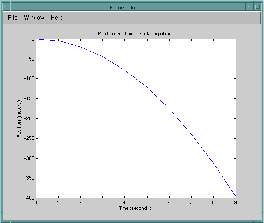## Call MATLAB Functions from C Applications

The program `engdemo.c` in the `matlabroot``/extern/examples/eng_mat` folder illustrates how to call the engine functions from a standalone C program. This example uses the C Matrix API.

Note

For calling MATLAB® functions from C++ applications, use the MATLAB Data API. For more information, see Call MATLAB Functions from C++.

For a Microsoft® Windows® version of this program, open `engwindemo.c` in the `matlabroot``\extern\examples\eng_mat` folder. For a C++ version, open `engdemo.cpp`.

The first part of this program starts MATLAB and sends it data. MATLAB analyzes the data and plots the results.The program continues with:

`Press Return to continue`

Pressing Return continues the program:

```Done for Part I. Enter a MATLAB command to evaluate. This command should create a variable X. This program will then determine what kind of variable you created. For example: X = 1:5```

Entering `X = 17.5` continues the program execution.

```X = 17.5 X = 17.5000 Retrieving X... X is class double Done!```

Finally, the program frees memory, closes the MATLAB engine, and exits.